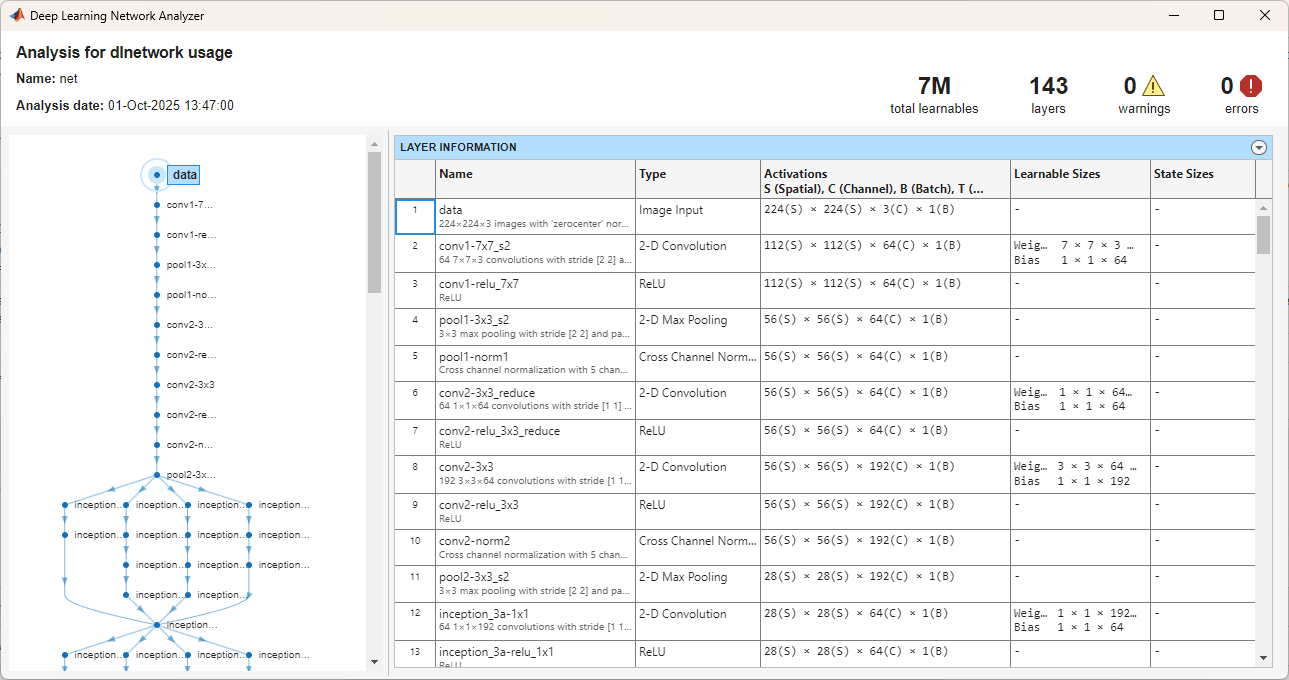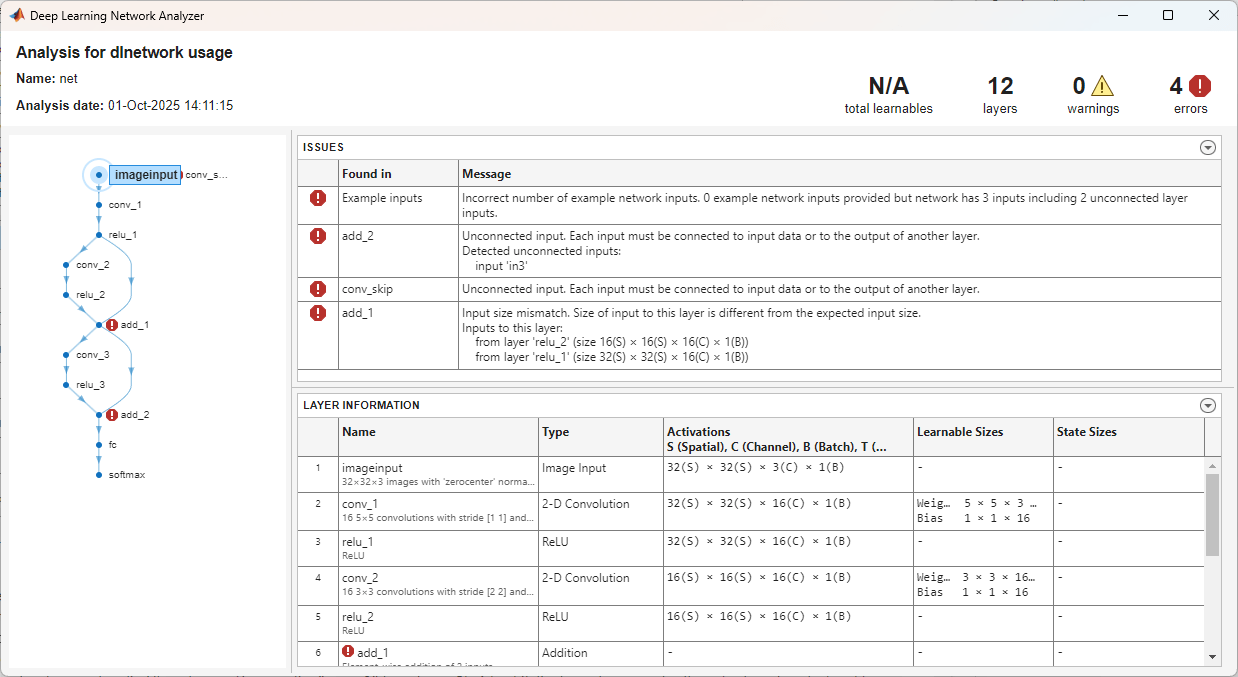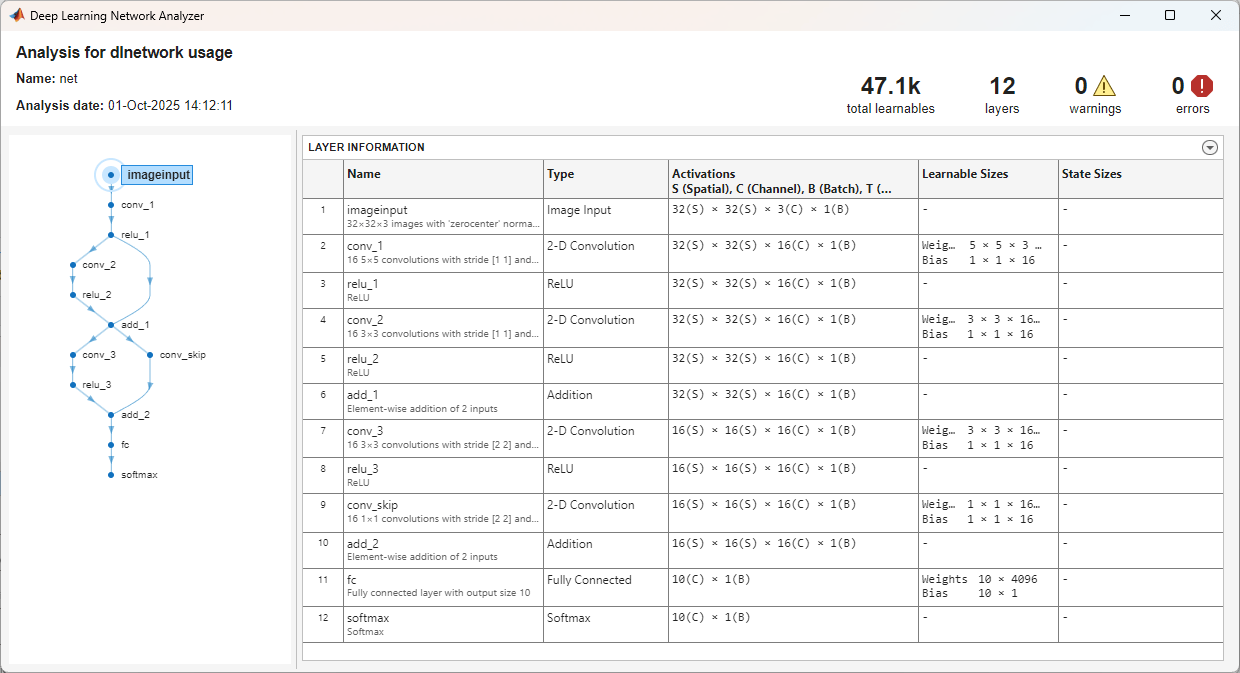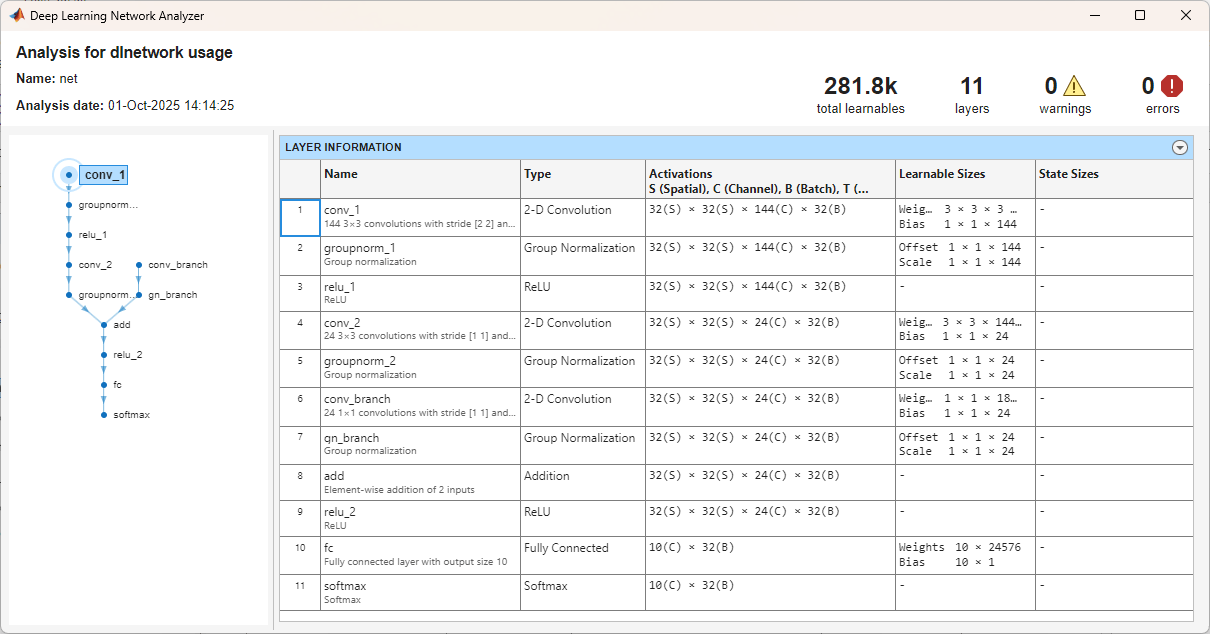# analyzeNetwork

Analyze deep learning network architecture

## Syntax

``analyzeNetwork(net)``
``analyzeNetwork(layers)``
``analyzeNetwork(layers,'TargetUsage',target)``
``analyzeNetwork(layers,dlX1,...,dlXn,'TargetUsage','dlnetwork')``
``analyzeNetwork(dlnet)``
``analyzeNetwork(dlnet,dlX1,...,dlXn)``

## Description

Use `analyzeNetwork` to visualize and understand the architecture of a network, check that you have defined the architecture correctly, and detect problems before training. Problems that `analyzeNetwork` detects include missing or unconnected layers, incorrectly sized layer inputs, an incorrect number of layer inputs, and invalid graph structures.

Tip

To interactively visualize, analyze, and train a network, use `deepNetworkDesigner(net)`. For more information, see Deep Network Designer.

### Trained Networks

example

````analyzeNetwork(net)` analyzes the `SeriesNetwork` or `DAGNetwork` object `net`. The function displays an interactive visualization of the network architecture and provides detailed information about the network layers. The layer information includes the number and sizes of layer activations, learnable parameters, and state parameters.```

### Network Layers

example

````analyzeNetwork(layers)` analyzes the network layers specified in `layers` and also detects errors and issues for `trainNetwork` workflows. `layers` can be a `Layer` array or a `LayerGraph` object. The function displays an interactive visualization of the network architecture and provides detailed information about the network layers. The layer information includes the number and sizes of layer activations, learnable parameters, and state parameters.```

example

````analyzeNetwork(layers,'TargetUsage',target)` analyzes the network layers specified in `layers` for the specified target workflow. Use this syntax when analyzing a `Layer` array or layer graph for `dlnetwork` workflows.```
````analyzeNetwork(layers,dlX1,...,dlXn,'TargetUsage','dlnetwork')` analyzes the network layers using example networks inputs `dlX1,...,dlXn`. The software propagates the example inputs through the network to determine the number and sizes of layer activations, learnable parameters, and state parameters. Use this syntax to analyze a network that has one or more inputs that are not connected to an input layer. ```

### `dlnetwork` Objects

````analyzeNetwork(dlnet)` analyzes the `dlnetwork` object for custom training loop workflows. The function displays an interactive visualization of the network architecture and provides detailed information about the network layers. The layer information includes the number and sizes of layer activations, learnable parameters, and state parameters.```
````analyzeNetwork(dlnet,dlX1,...,dlXn)` analyzes the `dlnetwork` object using example networks inputs `dlX1,...,dlXn`. The software propagates the example inputs through the network to determine the number and sizes of layer activations, learnable parameters, and state parameters. Use this syntax to analyze an uninitialized `dlnetwork` that has one or more inputs that are not connected to an input layer. ```

## Examples

collapse all

`net = googlenet`
```net = DAGNetwork with properties: Layers: [144×1 nnet.cnn.layer.Layer] Connections: [170×2 table] InputNames: {'data'} OutputNames: {'output'} ```

Analyze the network. `analyzeNetwork` displays an interactive plot of the network architecture and a table containing information about the network layers.

Investigate the network architecture using the plot to the left. Select a layer in the plot. The selected layer is highlighted in the plot and in the layer table.

In the table, view layer information such as layer properties, layer type, and sizes of the layer activations and learnable parameters. The activations of a layer are the outputs of that layer.

Select a deeper layer in the network. Notice that activations in deeper layers are smaller in the spatial dimensions (the first two dimensions) and larger in the channel dimension (the last dimension). Using this structure enables convolutional neural networks to gradually increase the number of extracted image features while decreasing the spatial resolution.

Show the total number of learnable parameters in each layer by clicking the arrow in the top-right corner of the layer table and select Total Learnables. To sort the layer table by column value, hover the mouse over the column heading and click the arrow that appears. For example, you can determine which layer contains the most parameters by sorting the layers by the total number of learnable parameters.

`analyzeNetwork(net)`Create a simple convolutional network with shortcut connections. Create the main branch of the network as an array of layers and create a layer graph from the layer array. `layerGraph` connects all the layers in `layers` sequentially.

```layers = [ imageInputLayer([32 32 3]) convolution2dLayer(5,16,'Padding','same') reluLayer('Name','relu_1') convolution2dLayer(3,16,'Padding','same','Stride',2) reluLayer additionLayer(2,'Name','add1') convolution2dLayer(3,16,'Padding','same','Stride',2) reluLayer additionLayer(3,'Name','add2') fullyConnectedLayer(10) softmaxLayer classificationLayer]; lgraph = layerGraph(layers);```

Create the shortcut connections. One of the shortcut connections contains a single 1-by-1 convolutional layer `skipConv`.

```skipConv = convolution2dLayer(1,16,'Stride',2,'Name','skipConv'); lgraph = addLayers(lgraph,skipConv); lgraph = connectLayers(lgraph,'relu_1','add1/in2'); lgraph = connectLayers(lgraph,'add1','add2/in2');```

Analyze the network architecture. `analyzeNetwork` finds four errors in the network.

`analyzeNetwork(lgraph)`Investigate and fix the errors in the network. In this example, the following issues cause the errors:

• The `skipConv` layer is not connected to the rest of the network. It should be a part of the shortcut connection between the `add1` and `add2` layers. To fix this error, connect `add1` to `skipConv` and `skipConv` to `add2`.

• The `add2` layer is specified to have three inputs, but the layers only has two inputs. To fix the error, specify the number of inputs as `2`.

• All the inputs to an addition layer must have the same size, but the `add1` layer has two inputs with different sizes. Because the `conv_2` layer has a `'Stride'` value of 2, this layer downsamples the activations by a factor of two in the first two dimensions (the spatial dimensions). To resize the input from the `relu2` layer so that it has the same size as the input from `relu1`, remove the downsampling by setting the `'Stride'` value of the `conv_2` layer to 1.

Apply these modifications to the layer graph construction from the beginning of this example and create a new layer graph.

```layers = [ imageInputLayer([32 32 3]) convolution2dLayer(5,16,'Padding','same') reluLayer('Name','relu_1') convolution2dLayer(3,16,'Padding','same','Stride',1) reluLayer additionLayer(2,'Name','add1') convolution2dLayer(3,16,'Padding','same','Stride',2) reluLayer additionLayer(2,'Name','add2') fullyConnectedLayer(10) softmaxLayer classificationLayer]; lgraph = layerGraph(layers); skipConv = convolution2dLayer(1,16,'Stride',2,'Name','skipConv'); lgraph = addLayers(lgraph,skipConv); lgraph = connectLayers(lgraph,'relu_1','add1/in2'); lgraph = connectLayers(lgraph,'add1','skipConv'); lgraph = connectLayers(lgraph,'skipConv','add2/in2');```

Analyze the new architecture. The new network does not contain any errors and is ready to be trained.

`analyzeNetwork(lgraph)`Create a layer graph for a custom training loop. For custom training loop workflows, the layer graph must not have an output layer.

```layers = [ imageInputLayer([28 28 1],'Normalization','none','Name','input') convolution2dLayer(5, 20,'Name','conv1') batchNormalizationLayer('Name','bn1') reluLayer('Name','relu1') convolution2dLayer(3,20,'Padding',1,'Name','conv2') batchNormalizationLayer('Name','bn2') reluLayer('Name','relu2') convolution2dLayer(3, 20,'Padding', 1,'Name','conv3') batchNormalizationLayer('Name','bn3') reluLayer('Name','relu3') fullyConnectedLayer(10,'Name','fc') softmaxLayer('Name','softmax')]; lgraph = layerGraph(layers);```

Analyze the layer graph using the `analyzeNetwork` function and set the `'TargetUsage'` option to `'dlnetwork'`.

`analyzeNetwork(lgraph,'TargetUsage','dlnetwork')`Here, the function does not report any issues with the layer graph.

To analyze a network that has an input that is not connected to an input layer, you can provide example network inputs to the `analyzeNetwork` function. You can provide example inputs when you analyze `dlnetwork` objects, or when you analyze `Layer` arrays or `LayerGraph` objects for custom training workflows using the `'TargetUsage','dlnetwork'` name-value option.

Define the network architecture. Construct a network with two branches. The network takes two inputs, with one input per branch. Connect the branches using an addition layer.

```numFilters = 24; inputSize = [64 64 3]; layersBranch1 = [ imageInputLayer(inputSize,'Normalization','none','Name','input') convolution2dLayer(3,6*numFilters,'Padding','same','Stride',2,'Name','conv1Branch1') groupNormalizationLayer('all-channels','Name','gn1Branch1') reluLayer('Name','relu1Branch1') convolution2dLayer(3,numFilters,'Padding','same','Name','conv2Branch1') groupNormalizationLayer('channel-wise','Name','gn2Branch1') additionLayer(2,'Name','add') reluLayer('Name','reluCombined') fullyConnectedLayer(10,'Name','fc') softmaxLayer('Name','sm')]; layersBranch2 = [ convolution2dLayer(1,numFilters,'Name','convBranch2') groupNormalizationLayer('all-channels','Name','gnBranch2')]; lgraph = layerGraph(layersBranch1); lgraph = addLayers(lgraph,layersBranch2); lgraph = connectLayers(lgraph,'gnBranch2','add/in2'); ```

Create the `dlnetwork`. Because this network contains an unconnected input, create an uninitialized `dlnetwork` object by setting the `'Initialize'` name-value option to `false`.

`dlnet = dlnetwork(lgraph,'Initialize',false);`

Create example network inputs of the same size and format as typical inputs for this network. For both inputs, use a batch size of 32. Use an input of size 64-by-64 with three channels for the input to the layer `'input'`. Use an input of size 64-by-64 with 18 channels for the input to the layer `'convBranch2'`.

```exampleInput = dlarray(rand([inputSize 32]),'SSCB'); exampleConvBranch2 = dlarray(rand([32 32 18 32]),'SSCB');```

Examine the `Layers` property of the network to determine the order in which to supply the example inputs.

`dlnet.Layers`
```ans = 12×1 Layer array with layers: 1 'input' Image Input 64×64×3 images 2 'conv1Branch1' Convolution 144 3×3 convolutions with stride [2 2] and padding 'same' 3 'gn1Branch1' Group Normalization Group normalization 4 'relu1Branch1' ReLU ReLU 5 'conv2Branch1' Convolution 24 3×3 convolutions with stride [1 1] and padding 'same' 6 'gn2Branch1' Group Normalization Group normalization 7 'add' Addition Element-wise addition of 2 inputs 8 'reluCombined' ReLU ReLU 9 'fc' Fully Connected 10 fully connected layer 10 'sm' Softmax softmax 11 'convBranch2' Convolution 24 1×1 convolutions with stride [1 1] and padding [0 0 0 0] 12 'gnBranch2' Group Normalization Group normalization ```

Analyze the network. Provide the example inputs in the same order as the layers that require inputs appear in the `Layers` property of the `dlnetwork`. You must provide an example input for all network inputs, including inputs that are connected to an input layer.

`analyzeNetwork(dlnet,exampleInput,exampleConvBranch2)`## Input Arguments

collapse all

Trained network, specified as a `SeriesNetwork` or a `DAGNetwork` object. You can get a trained network by importing a pretrained network (for example, by using the `googlenet` function) or by training your own network using `trainNetwork`.

Network layers, specified as a `Layer` array or a `LayerGraph` object.

For a list of built-in layers, see List of Deep Learning Layers.

Network for custom training loops, specified as a `dlnetwork` object.

Target workflow, specified as one of the following:

• `'trainNetwork'` — Analyze layer graph for usage with the `trainNetwork` function. For example, the function checks that the layer graph has an output layer and no disconnected layer outputs.

• `'dlnetwork'` — Analyze layer graph for usage with `dlnetwork` objects. For example, the function checks that the layer graph does not have any output layers.

Example network inputs, specified as formatted `dlarray` objects. The software propagates the example inputs through the network to determine the number and sizes of layer activations, learnable parameters, and state parameters.

Use example inputs when you want to analyze a network that has inputs that are unconnected to an input layer.

The order in which you must specify the example inputs depends on the type of network you are analyzing:

• `Layer` array — Provide example inputs in the same order that the layers that require inputs appear in the `Layer` array.

• `LayerGraph` — Provide example inputs in the same order as the layers that require inputs appear in the `Layers` property of the `LayerGraph`.

• `dlnetwork` — Provide example inputs in the same order as the inputs are listed in the `InputNames` property of the `dlnetwork`.

If a layer has multiple unconnected inputs, then example inputs for that layer must be specified separately in the same order as they appear in the layer’s `InputNames` property.

You must specify one example input for each input to the network, even if that input is connected to an input layer.

Introduced in R2018a